Get inspired by the success stories of our students in IIT JAM MS, ISI  MStat, CMI MSc DS.  Learn More

# ISI MStat PSB 2005 Problem 3 | The Orthogonal MatrixThis is a very subtle sample problem from ISI MStat PSB 2005 Problem 3. Given that one knows the property of orthogonal matrices its just a counting problem. Give it a thought!

## Problem- ISI MStat PSB 2005 Problem 3

Let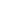be a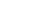orthogonal matrix, where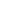is even and suppose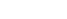, where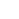denotes the determinant of. Show that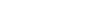, where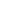denotes theidentity matrix.

### Prerequisites

Orthogonal Matrix

Eigenvalues

Characteristic Polynomial

## Solution :

This is a very simple problem, when you are aware of the basic facts.

We, know that, the eigenvalues of a orthogonal matrix is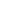and.(and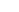if its skew-symmetric). But this given matrixis not skew-symmetric.(Why??).So let for the matrix, the algebraic multiplicity ofandbe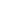and, respectively.

So, since, hence the algebraic multiplicity ofis definitely odd, since we know by the property of eigenvalues determinant of a matrix is just the product of its eigenvalues.

Now since,is even and the algebraic multiplicity ofi.e.is odd, henceis also odd and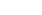.

Hence, the Characteristic Polynomial of, is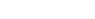, where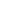is the eigenvalue of, and in this problem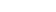or.

Hence, putting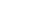, we conclude that,. Hence we are done !!

## Food For Thought

Now, supposeis any non-singular matrix, such that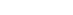. What can you say about the column space of?

Keep thinking !!

## Subscribe to Cheenta at Youtube

This is a very subtle sample problem from ISI MStat PSB 2005 Problem 3. Given that one knows the property of orthogonal matrices its just a counting problem. Give it a thought!

## Problem- ISI MStat PSB 2005 Problem 3

Letbe aorthogonal matrix, whereis even and suppose, wheredenotes the determinant of. Show that, wheredenotes theidentity matrix.

### Prerequisites

Orthogonal Matrix

Eigenvalues

Characteristic Polynomial

## Solution :

This is a very simple problem, when you are aware of the basic facts.

We, know that, the eigenvalues of a orthogonal matrix isand.(andif its skew-symmetric). But this given matrixis not skew-symmetric.(Why??).So let for the matrix, the algebraic multiplicity ofandbeand, respectively.

So, since, hence the algebraic multiplicity ofis definitely odd, since we know by the property of eigenvalues determinant of a matrix is just the product of its eigenvalues.

Now since,is even and the algebraic multiplicity ofi.e.is odd, henceis also odd and.

Hence, the Characteristic Polynomial of, is, whereis the eigenvalue of, and in this problemor.

Hence, putting, we conclude that,. Hence we are done !!

## Food For Thought

Now, supposeis any non-singular matrix, such that. What can you say about the column space of?

Keep thinking !!

## Subscribe to Cheenta at Youtube

This site uses Akismet to reduce spam. Learn how your comment data is processed.

### Knowledge Partner